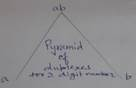Squaring Any Number Using Duplex Pyramid Method

H.D. Motiramani

Squaring any number with Duplex Pyramid method is very easy.

First we consider a two digit square say ab² where ‘a’ and ‘b’ represent the two digits.

As shown in figure below Pyramid looks like a triangle with left hand corner as duplex of ‘a’, right hand corner as duplex of ‘b’ and the top as duplex of ‘ab’.

And the answer to the problem ab² will be duplex of a/ duplex of ab / duplex of b

When we say duplex, let us know that Duplex of ‘a’ (a single digit) is a², similarly duplex of single digit ‘b’ will be b² and duplex of ‘ab’ will be twice the multiplication of ‘a’ and ‘b’. Therefore, ab² will be equal to a²I 2ab I b²

The pyramid will look like thisDuplex of a= a², Duplex of ab= 2×a×b, Duplex of b=b²

If now square of say 47 is intended to be found out, 4 and 7 can be looked as ‘a’ and ‘b’

Therefore, the answer will be 4²/2×4×7/7² or 16/56/49

Now comes the part of addition. Addition starts from right. From 49in the right portion, 9 can be retained and 4 carried over to left to be added to 56. 56 will become 60. We will retain 0 of 60 and carry over 6 to be added to 16 to make it 22. So the answer will be 2209

Examples:

43² =       4² I 2× 4× 3I 3²    =    16 I     24     I 9     =        18  4  I 9           = 1849

78² =       7² I 2× 7× 8I 8²    =     49I   112    I 64     =      60 I    8  I 4          =  6084

87² =       8²I 2× 8× 7 I 7²    =      8²I 2×8×7 I 7²     =       64 I 112 I 49        =  7569

For a three digit number to be squared, say ‘abc’, the pyramid from left bottom will be duplex of  ‘a’in the bottom left followed by duplex of ‘ab’ in the middle, duplex of abc at top, duplex of bc in the middle of sloping part on right and finally duplex of c at the right hand bottom. When we talk of duplex of a three digit number, it will be equal to middle digit square plus twice the multiplication of 1st and last digit of that three digit number. So duplex of ‘abc’ will be b²+ 2ac. For say duplex of 123 it will be 2²+2×1×3=10Duplex of a= a², Duplex of ab=2×a×b,Duplex of abc= b²+2×a×c,Duplex of bc=2×b×c, Duplex of c=c²

Examples:

347²     = duplex of 3   I duplex of 34   I duplex of 347         I duplex of 47 I  duplex of 7

=        3²           I        2×3×4      I    4²+2×3×7            I      2×4×7      I         7²

=         9           I           24          I              58              I         56         I        49        =  120409

234²      =        4             I            12         I             25              I        24           I     16          =    54756

456²      =     16              I          40           I             73              I        60            I   36          =  207936

—00—

<